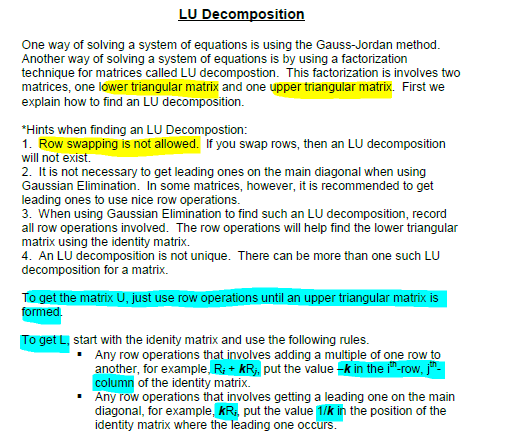# Checking Correctness of LU Decomposition

• twoski

## Homework Statement

compute the LU decomposition of the 3x3 matrix:

A= 2, 1, 1/2
1/2, 2, 1
1, 1/2, 2

Let f be the vector (5,3,6)

Solve Lg=f and then Ux=g. You can check your answer with $A_{3}x=f$

## The Attempt at a Solution

I finished the calculations:

U = 1, 1/2, 1/4
0, 1, 1/2
0, 0, 1

L = 1, 0, 0
-1/4, 1, 0
-1/2, 0, 1

g = (5, 17/4, 17/2)
f = (23/8, 0, 17/2)

I want to check my answer. What does the subscript 3 signify?

Probably a typo.

Hm, okay, so if that's a typo then checking is as easy as doing Ax=f?

When i check, i end up with (10, 159/16, 159/8) which is certainly wrong.

I am trying to follow some instructions on LU decomposition because our professor's notes are really lacking, and it says:

To get L, start with the idenity matrix and use the following rules. Any row operations that involves adding a multiple of one row to another, for example, Ri + kRj, put the value –k in the ith-row, jth-column of the identity matrix.

Any row operations that involves getting a leading one on the main diagonal, for example, kRi, put the value 1/k in the position of the identity matrix where the leading one occurs.

So, if i did 1/2R1 when working on my U matrix, would i multiply the leading 1 in R1 by 2 when getting my L matrix? I keep trying to recalculate the g vector and every time i end up with very specific fractions like 19/14 which seems wrong to me. The vector f has no fractions in it so ideally my x vector shouldn't contain any weird fractions.

Last edited:
First and foremost, A = LU. I don't think you have the correct decomposition, certainly not by trying to calculate a11.

I followed these directions exactly and I don't even end up with proper L and U matrices. How frustrating.If A =
2, 1, 1/2
1/2, 2, 1
1, 1/2, 2

Then all I need to do is:

R2-1/4R1
R3-1/2R1
1/2R1
4/7R2
4/7R3

Now according to the notes, i have to use these as a reference for computing L.

A2,1 = 1/4
A3,1 = 1/2
A1,1 = 2
A2,2 = 7/4
A3,3 = 7/4

Am i doing anything wrong?

The instructions you're following are flawed or incomplete.

Each row operation can be represented by a matrix. For example, R2 = R2-1/4 R1 can be represented by the lower-triangular matrix
$$O_1 = \begin{pmatrix} 1 & 0 & 0 \\ -\frac{1}{4} & 1 & 0 \\ 0 & 0 & 1 \end{pmatrix}.$$ If you multiply A on the left by O1, you'll see you get the result of performing the row operation. So you did a bunch of row operations to get the upper triangular matrix. In terms of the matrices, you have
$$U = O_n O_{n-1} \dots O_2 O_1 A.$$ Each of the row operations is invertible, so its corresponding matrix is invertible. That means you can multiply by the inverses to get
$$O_1^{-1} O_2^{-1}\dots O_{n-1}^{-1}O_n^{-1} U = A.$$ The product of the inverses gives you L.

The instructions you were given tell you how to write down the inverses for each row operation. For instance, if you invert O1, you'd get
$$O_1^{-1} = \begin{pmatrix} 1 & 0 & 0 \\ \frac{1}{4} & 1 & 0 \\ 0 & 0 & 1\end{pmatrix}.$$ So rather than try to write down L in one fell swoop as you've tried to do, you want to write down the five inverse matrices separately, and then multiply them together in the correct order to get L.